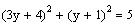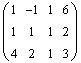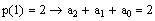then,Let
5. Find a polynomial that passes through the points (-1, 6), (1, 2), (2, 3). Solve the system by computing the reduced row echelon form of the augmented matrix.or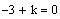The system will have a unique solution, i.e., the lines will intersect at a point if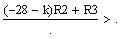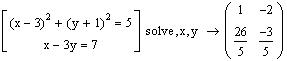4. Find the value of k for which the three straight lines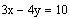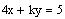intersect at a point on the plane.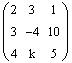Form the augmented matrix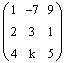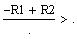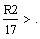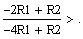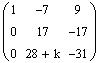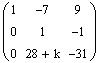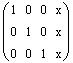d) 3 equations in 3 unknowns that has a unique solution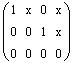c) 3 equations in 3 unknowns that has infinite number of solutions
Not possible because the maximum rank is 3 and the number of unknowns is 4.
b) 3 equations in 4 unknowns that has a unique solution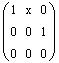a) 3 equations in 2 unknowns that has no solution
6. Extra Credit: If possible, give examples of the reduced row echelon form of the augmented matrix of a linear system with the given property. If not possible, write "Not Possible" as an answer. Use " * " to indicate that an element could be any real number.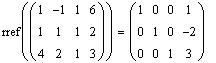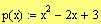The polynomial is:
Form the augmented matrix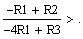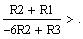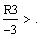This is the reduced row echelon form of the augmented matrix.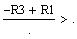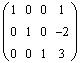Thus,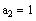and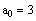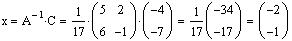c) Use the inverse of A to find the solution of the system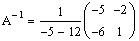b) Compute the inverse of the coefficient matrix A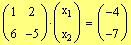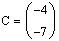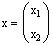a) Write the system in matrix form Ax = C2. Given the system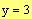and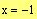Math 002-042
Major Quiz 3
May 25, 2005
Name:
Student ID:
Section #.:09
List #.:
1. Solve for x and y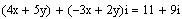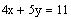Equate the real and imaginary parts of the two sides of the equation
==>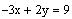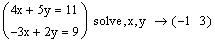Thus,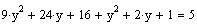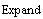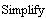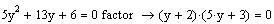Divide by 2 then factor
==>or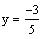Substitute in (3)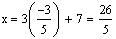Thus, the solutions areandThus,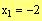and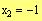3. Solve the nonlinear system(1)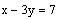(2)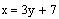(3)
Solve for xSubstitute in (1)